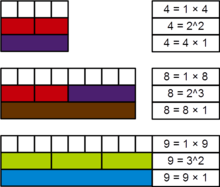# Perfect powerDemonstration, with Cuisenaire rods, of the perfect power nature of 4, 8, and 9

In mathematics, a perfect power is a positive integer that can be expressed as an integer power of another positive integer. More formally, n is a perfect power if there exist natural numbers m > 1, and k > 1 such that mk = n. In this case, n may be called a perfect kth power. If k = 2 or k = 3, then n is called a perfect square or perfect cube, respectively. Sometimes 1 is also considered a perfect power (1k = 1 for any k).

## Examples and sums

A sequence of perfect powers can be generated by iterating through the possible values for m and k. The first few ascending perfect powers in numerical order (showing duplicate powers) are (sequence A072103 in the OEIS):The sum of the reciprocals of the perfect powers (including duplicates) is 1:which can be proved as follows:The first perfect powers without duplicates are:

(sometimes 0 and 1), 4, 8, 9, 16, 25, 27, 32, 36, 49, 64, 81, 100, 121, 125, 128, 144, 169, 196, 216, 225, 243, 256, 289, 324, 343, 361, 400, 441, 484, 512, 529, 576, 625, 676, 729, 784, 841, 900, 961, 1000, 1024, ... (sequence A001597 in the OEIS)

The sum of the reciprocals of the perfect powers p without duplicates is:where μ(k) is the Möbius function and ζ(k) is the Riemann zeta function.

According to Euler, Goldbach showed (in a now lost letter) that the sum of 1/(p−1) over the set of perfect powers p, excluding 1 and excluding duplicates, is 1:This is sometimes known as the Goldbach-Euler theorem.

## Detecting perfect powers

Detecting whether or not a given natural number n is a perfect power may be accomplished in many different ways, with varying levels of complexity. One of the simplest such methods is to consider all possible values for k across each of the divisors of n, up to. So if the divisors ofarethen one of the valuesmust be equal to n if n is indeed a perfect power.

This method can immediately be simplified by instead considering only prime values of k. This is because iffor a compositewhere p is prime, then this can simply be rewritten as. Because of this result, the minimal value of k must necessarily be prime.

If the full factorization of n is known, saywhere theare distinct primes, then n is a perfect power if and only ifwhere gcd denotes the greatest common divisor. As an example, consider n = 296·360·724. Since gcd(96, 60, 24) = 12, n is a perfect 12th power (and a perfect 6th power, 4th power, cube and square, since 6, 4, 3 and 2 divide 12).

## Gaps between perfect powers

In 2002 Romanian mathematician Preda Mihăilescu proved that the only pair of consecutive perfect powers is 23 = 8 and 32 = 9, thus proving Catalan's conjecture.

Pillai's conjecture states that for any given positive integer k there are only a finite number of pairs of perfect powers whose difference is k. This is an unsolved problem.

## Calculation by recursion for positive integers

As an alternate way to calculate perfect powers, the recursive approach has yet to be found useful. It is based on the observation that the difference between ab and (a+1)b wheremay not be constant, but if you take the difference of successive differences, b times, there is a constant b! factor. For example, 94 = 6561, and 104 is 10000. the difference is 3439. The difference between 84 and 94 is 2465, meaning the difference of differences is 974. A step further and you have 204. One step further, and you have 24, which is equal to 4!. One step further and collating this 'key' row from progressively larger exponents yields a triangle similar to Pascal's, but with a differing formula for generation. A part of this table is shown below:

Define the following function on the range of positive integers:where a = 1 or a = bwhere b > aelsewhere

This function generates the following output:

1 2 3 4 5 6
1 1 0 0 0 0 0
2 1 1 0 0 0 0
3 1 4 1 0 0 0
4 1 11 11 1 0 0
5 1 26 66 26 1 0
6 1 57 302 302 57 1

Also define the following function on the range of positive integers: (This is very closely related to the Binomial Theorem and Pascal's Triangle)where a = 1 or b = 1elsewhere

The table this generates can be seen as pascal's triangle fallen over to the left, so that what were rows on Pascal's triangle have become diagonal series in the table.

1 2 3 4 5 6 7 8
1 1 1 1 1 1 1 1 1
2 1 2 3 4 5 6 7 8
3 1 3 6 10 15 21 28 36
4 1 4 10 20 35 56 84 120
5 1 5 15 35 70 126 210 330
6 1 6 21 56 126 252 462 792
7 1 7 28 84 210 462 924 1716
8 1 8 36 120 330 792 1716 3432

It can then be stated that:Example:Expanding P(7,4)Or you can look up the values on the table and get P(6,4) = 56, and P(5,4) = 35.

By definition, K(3,1) = 1. Expanding K(3,2)By definition, K(3,3) = 1.This calculation method can be used for all integer power calculations, as negative integers act the same way, simply applying the negative if the exponent is odd.